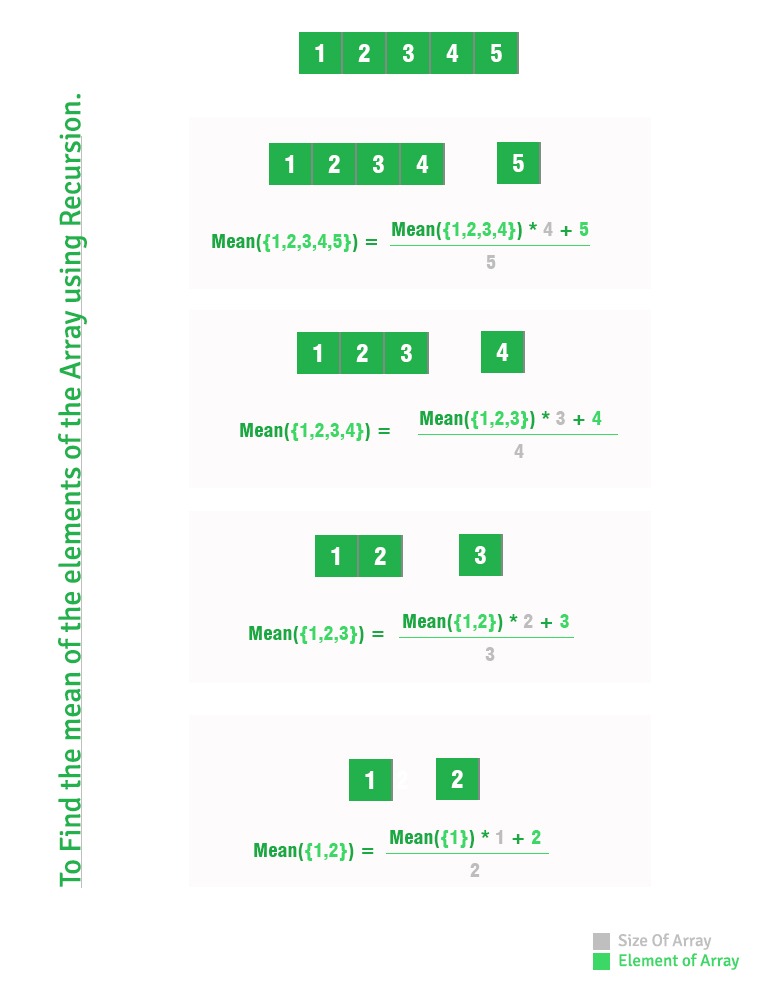Open in App
Not now

# Mean of array using recursion

• Difficulty Level : Easy
• Last Updated : 16 Feb, 2023

To find the mean of the elements of the array.

```Mean = (Sum of elements of the Array) /
(Total no of elements in Array)```

Examples:

```Input : 1 2 3 4 5
Output : 3

Input : 1 2 3
Output : 2```

To find the mean using recursion assume that the problem is already solved for N-1 ie you have to find for n

```Sum of first N-1 elements =
(Mean of N-1 elements)*(N-1)

Mean of N elements = (Sum of first N-1 elements +
N-th elements) / (N)```

Note : Since array indexing starts from 0, we access N-th element using A[N-1].Implementation:

## C++

 `// Recursive C++ program to find mean of array``#include ``using` `namespace` `std;`` ` `// Function Definition of findMean function``float` `findMean(``int` `A[], ``int` `N)``{``    ``if` `(N == 1)``        ``return` `(``float``)A[N-1];``    ``else``        ``return` `((``float``)(findMean(A, N-1)*(N-1) +``                                    ``A[N-1]) / N);``}`` ` `// Main Calling Function``int` `main()``{``    ``float` `Mean = 0;``    ``int` `A[] = {1, 2, 3, 4, 5};``    ``int` `N = ``sizeof``(A)/``sizeof``(A);``    ``cout << ``" "``<< findMean(A, N);``    ``return` `0;``}`` ` `// this code is contributed by shivanisinghss2110`

## C

 `// Recursive C program to find mean of array``#include`` ` `// Function Definition of findMean function``float` `findMean(``int` `A[], ``int` `N)``{``    ``if` `(N == 1)``        ``return` `(``float``)A[N-1];``    ``else``        ``return` `((``float``)(findMean(A, N-1)*(N-1) +``                                    ``A[N-1]) / N);``}`` ` `// Main Calling Function``int` `main()``{``    ``float` `Mean = 0;``    ``int` `A[] = {1, 2, 3, 4, 5};``    ``int` `N = ``sizeof``(A)/``sizeof``(A);``    ``printf``(``"%.2f\n"``, findMean(A, N));``    ``return` `0;``}`

## Java

 `// Recursive Java program to find mean of array``import` `java.io.*;`` ` `class` `CalcMean``{``    ``// Function Definition of findMean function``    ``static` `float` `findMean(``int` `A[], ``int` `N)``    ``{``        ``if` `(N == ``1``)``            ``return` `(``float``)A[N-``1``];``        ``else``            ``return` `((``float``)(findMean(A, N-``1``)*(N-``1``) +``                                        ``A[N-``1``]) / N);``    ``}``     ` `    ``// main Function``    ``public` `static` `void` `main (String[] args) ``    ``{``        ``float` `Mean = ``0``;``        ``int` `A[] = {``1``, ``2``, ``3``, ``4``, ``5``};``        ``int` `N = A.length;``        ``System.out.println(findMean(A, N));``    ``}``}`

## Python3

 `# Recursive Python3 program to``# find mean of array`` ` `# Function Definition of findMean function``def` `findMean(A, N):`` ` `    ``if` `(N ``=``=` `1``):``        ``return` `A[N ``-` `1``]``    ``else``:``        ``return` `((findMean(A, N ``-` `1``) ``*``                ``(N ``-` `1``) ``+` `A[N ``-` `1``]) ``/` `N)`` ` `# Driver Code``Mean ``=` `0``A ``=` `[``1``, ``2``, ``3``, ``4``, ``5``]``N ``=` `len``(A)``print``(findMean(A, N))`` ` `# This code is contributed by Anant Agarwal.`

## C#

 `// Recursive C# program to find mean of array``using` `System;`` ` `class` `CalcMean``{``    ``// Function Definition of findMean function``    ``static` `float` `findMean(``int` `[]A, ``int` `N)``    ``{``        ``if` `(N == 1)``            ``return` `(``float``)A[N - 1];``        ``else``            ``return` `((``float``)(findMean(A, N - 1) * ``                           ``(N - 1) + A[N - 1]) / N);``    ``}``      ` `    ``// Driver code``    ``public` `static` `void` `Main() ``    ``{``        ``//float Mean = 0;``        ``int` `[]A = {1, 2, 3, 4, 5};``        ``int` `N = A.Length;``        ``Console.WriteLine(findMean(A, N));``    ``}``}`` ` `// This code is contributed by Anant Agarwal.`

## PHP

 ``

## Javascript

 ``

Output

` 3`

Time Complexity: O(n)
Auxiliary Space: O(n)

This article is contributed by Prakhar Agrawal. If you like GeeksforGeeks and would like to contribute, you can also write an article using write.geeksforgeeks.org or mail your article to review-team@geeksforgeeks.org. See your article appearing on the GeeksforGeeks main page and help other Geeks.

My Personal Notes arrow_drop_up